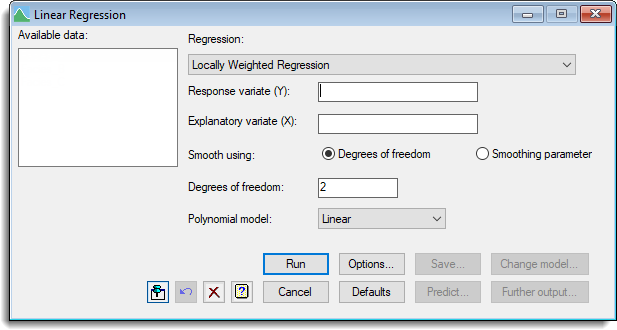1. Home
2. Linear Regression

# Linear Regression

Select menu: Stats | Regression Analysis | Linear Models

The Linear Regression dialog allows a variety of linear regression models to be fitted, selected from those listed in the Regression dropdown list.

Stats | Regression Analysis | Linear Models.
2. Fill in the fields as required then click Run.

You can set additional Options then after running, you can save the results by clicking Save.## Available data

This lists data structures appropriate to the current input field. The contents of this box will change as you move from one field to the next. You can double-click a name in the list box to copy it to the current input field.

## Regression

Selects the type of regression model to be analysed. See the help specific to each model for a description of its features and the information to be entered. These menus provide for simple and multiple linear regression, with or without groups, polynomial regression, smoothing splines and locally weighted regression. In addition, the general linear regression option lets you construct your own linear model, using linear, polynomial or smoothed effects of variates, main effects of factors, and interactions.

## Options

This controls display options, allowing you to select the output to be generated initially by the regression – the same information can also be printed after the analysis, using Further output. It also controls whether a constant term (or intercept) is included in the model.

## Further output

Lets you display additional results and graphical output from the analysis.

## Save

Lets you specify data structures in which to save fitted values, residuals, parameter estimates, and standard errors, variances and covariances of estimates from the analysis.

## Predict

Allows you to form predictions based on the current regression model using the Predictions dialog associated with the model being fitted:

## Change model

This changes the current model by adding or dropping explanatory terms, thus allowing a sequence of models to be fitted and assessed. The option is only available for the General linear regression model.